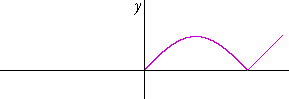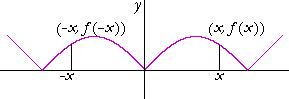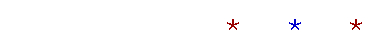Topics in

P R E C A L C U L U S

16

# SYMMETRY

Test for symmetry:  Even and odd functions

LET THIS BE THE RIGHT-HAND SIDE of the graph of a function:We will now draw the left-hand side -- so that the graph will be symmetrical with respect to the y-axis:In this case,

f(−x) = f(x).

The height of the curve at −x is equal to the height of the curve at x -- for every x in the domain of f.

Again, let this be the right-hand side:We will now draw the left-hand side -- so that the graph will be symmetrical with respect to the origin:Every point on the right-hand side is reflected through the origin.  In this case,

f(−x) = −f(x).

The value of f at −x is the negative of the value at x.

(A reflection through the origin is equivalent to a reflection about the y-axis, followed by a reflection about the x-axis.)

Test for symmetry: Even and odd functions

Symmetry, then, depends on the behavior of f(x) on the other side of the y-axis -- at minus-x :   f(−x).

Here is the test:

If f(−x) = f(x),

then the graph of f(x) is symmetrical with respect to the y-axis.

If f(−x) = −f(x),

then the graph of f(x) is symmetrical with respect to the origin.

A function symmetrical with respect to the y-axis is called an even function.

A function that is symmetrical with respect to the origin  is called an odd function.

Example 1.   Test this function for symmetry:

f(x) = x4 + x2 + 3

Solution.   We must look at f(−x):

 f(−x) = (−x)4 + (−x)2 + 3 = x4 + x2 + 3 = f(x).

Since  f(−x) = f(x), this function is symmetrical with respect to the y-axis. It is an even function.

Example 2.   Test this function for symmetry:

f(x) = x5 + x3 + x

Solution.   Again, we must look at f(−x):

 f(−x) = (−x)5 + (−x)3 + (−x) = −x5 − x3 − x = −(x5 + x3 + x) = −f(x).

Since  f(−x) = −f(x), this function is symmetrical with respect to the origin. It is an odd function.

Problem.   Test each of the following for symmetry.  Is f(x) even, odd, or neither?

a)  f(x) = x3 + x2 + x + 1

Answer.   Neither, because f(−x) ≠ f(x) , and f(−x) ≠ −f(x).

b)  f(x) = 2x3 − 4x

Answer.   f(x) is odd—it is symmetrical with respect to the origin—because f(−x) = −f(x).

c)  f(x) = 7x2 − 11

Answer.   f(x) is even—it is symmetrical with respect to the y-axis—because f(−x) = f(x).

Note:  A polynomial will be an even function when all the exponents are even.

A polynomial will be an odd function when all the exponents are odd.

But there are even and odd functions that are not polynomials. In trigonometry,  y = cos x is an even function, while y = sin x is odd.

Therefore, the issue is the test of f(−x).Next Topic:  Translations

Please make a donation to keep TheMathPage online.
Even \$1 will help.